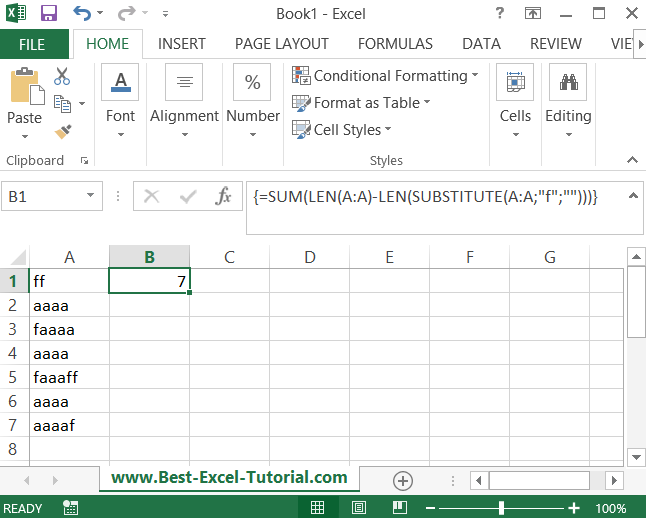#### Counting occurrences of a character in a spreadsheet

In this Excel tutorial you will teach yourself how to count occurences of a character in a spreadsheet.

## How to count occurrences of character in a string?

Let’s count how many of f character do you have in a A coulmn.

To do that just use below formula:

{=SUM(LEN(A:A)-LEN(SUBSTITUTE(A:A;”f”;””)))}

This is an array formula so remember to press CTRL + SHIFT + ENTER combination.Counting occurrences of a character in a spreadsheet may be useful for spreadsheets with unique text.

Also you may be interested in counting character which is related to your company/project and used as a your internal code.

## How to count cells with a specific content in a range?

In this example you will see how to count the number of cells with specific content in a range in clever way. You need only one formula to do this!

To count a number of cells with a text in a specific range you just need to use sumproduct function.

You can build formula like this:

=SUMPRODUCT(–ISTEXT(RANGE))

You can count a number of cells with a value as well with similar formula:

=SUMPRODUCT(–ISNUMBER(RANGE))

### Other examples

There are others functions you can use with this kind of formulas:

• =SUMPRODUCT(–ISBLANK(RANGE))
• =SUMPRODUCT(–ISERR(RANGE))
• =SUMPRODUCT(–ISERROR(RANGE))
• =SUMPRODUCT(–ISEVEN(NUMBER))
• =SUMPRODUCT(–ISFORMULA(RANGE))
• =SUMPRODUCT(–ISLOGICAL(RANGE))
• =SUMPRODUCT(–ISNA(RANGE))
• =SUMPRODUCT(–ISNONTEXT(RANGE))
• =SUMPRODUCT(–ISODD(NUMBER))
• =SUMPRODUCT(–ISOWEEKNUM(DATE))
• =SUMPRODUCT(–ISPMT(RATE,PER,NPER,PV))
• =SUMPRODUCT(–ISREF(RANGE))

## How to count occurrences using Vba?

In Excel VBA, you can count the number of occurrences of a certain value in a range using the following code:

Function CountOccurrences(rng As Range, value As Variant) As Long CountOccurrences = WorksheetFunction.CountIf(rng, value) End Function

You can then use this function in your VBA code by passing in the range you want to search and the value you want to count occurrences of. For example:

`Sub ExampleCountOccurrences()`

`  Dim rng As Range `

`  Set rng = Range("A1:A10") ' Define the range you want to search`

`  Dim value As Variant`

`  value = "apple" ' Define the value you want to count `

`  Dim count As Long`

`  count = CountOccurrences(rng, value) `

`MsgBox count & " occurrences of " & value & " found in range " & rng.Address `

`End Sub`

This code will return the number of occurrences of the value “apple” in the range “A1:A10”.

Isn’t an Excel wonderful that makes your life so much easier?